output.to from Sideway
Draft for Information Only

# Content

`Current Density and Conductivity Resistance Resistors in Series Resistors in Parallel Capacitor in Series Capacitor in Parallel Source and Reference`

# Current Density and Conductivity

The current density 𝐽 is the current per unit area 𝐴, ```𝐼=|𝑞|𝑛𝐴𝑣=|𝑞|𝑛𝐴𝑢𝐸 𝐽≡𝐼𝐴=|𝑞|𝑛𝐴𝑢𝐸𝐴=|𝑞|𝑛𝑢𝐸 ``` The conductivity 𝜎 is a mterial-dependent quantity. `𝐽=|𝑞|𝑛𝑢𝐸≡𝜎𝐸⇒𝜎=|𝑞|𝑛𝑢` Conductivity add, even for opposite sign charge carriers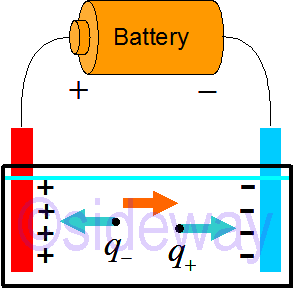That is `𝜎=|𝑞+|𝑛+𝑢++|𝑞−|𝑛−𝑢−`

## Resistance

The resistance of a resistor of length 𝐿, ```∆𝑉=−∫𝐸⋅𝑑𝑙⇒|∆𝑉|=𝐸𝐿 𝐼=𝑞𝑛𝐴𝑢𝐸=𝜎𝐴𝐸 𝐼=𝜎𝐴𝐸=𝜎𝐴|∆𝑉|𝐿⇒|∆𝑉|=𝐼𝐿𝜎𝐴 ∵|∆𝑉|=𝐼𝑅⇒𝑅=𝐿𝜎𝐴 ```

## Resistors in Series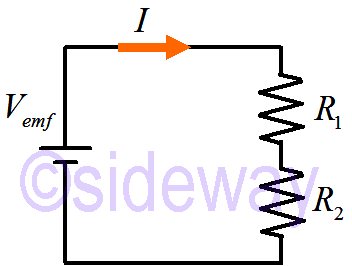When resistors are in series, the effective series resistance is ```𝐼=𝐼1=𝐼2 ∆𝑉emf+∆𝑉1+∆𝑉2=0 ⇒𝑉emf−𝐼𝑅1−𝐼𝑅2=0 ⇒𝑉emf=𝐼𝑅1+𝐼𝑅2=𝐼(𝑅1+𝑅2)=𝐼𝑅equivalent ⇒𝑅equivalent=𝑅1+𝑅2``` For resistor made of the same material and with the same 𝐴, the resistance follows straight from the definition of resistance 𝑅=𝐿𝜎𝐴 ```𝑅equivalent=𝐿1𝜎𝐴+𝐿2𝜎𝐴=𝐿1+𝐿2𝜎𝐴=𝐿equivalent𝜎𝐴 ⇒𝐿equivalent=𝐿1+𝐿2```

## Resistors in Parallel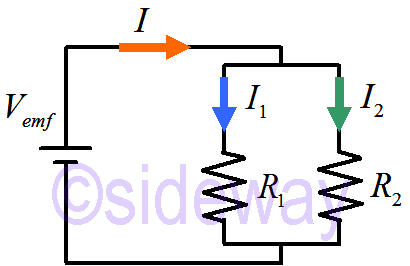When resistors are in parallel, the effective parallel resistance is ```𝐼=𝐼1+𝐼2=𝑉emf𝑅1+𝑉emf𝑅2=1𝑅1+1𝑅2𝑉emf=1𝑅equivalent𝑉emf ⇒1𝑅equivalent=1𝑅1+1𝑅2=𝑅1+𝑅1𝑅1𝑅1 ``` For resistor made of the same material and with the same 𝐿, the resistance follows straight from the definition of resistance 𝑅=𝐿𝜎𝐴 ```𝑅=𝐿𝜎𝐴⇒1𝑅=𝜎𝐴𝐿 ⇒1𝑅equivalent=𝜎𝐴1𝐿+𝜎𝐴1𝐿=𝜎𝐿(𝐴1+𝐴2)=𝜎𝐿𝐴equivalent ⇒𝐴equivalent=𝐴1+𝐴2 ```

## Capacitor in Series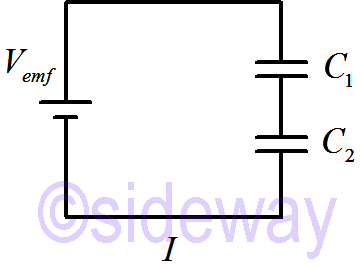When capacitors are in series, the effective series capacitance is ```𝐼=𝐼1=𝐼2⇒𝑄1=𝑄2=𝑄 ∆𝑉emf+∆𝑉1+∆𝑉2=0 ⇒𝑉emf−𝑄𝐶1−𝑄𝐶2=0 ⇒𝑉emf=𝑄𝐶1+𝑄𝐶2=𝑄1𝐶1+1𝐶2=𝑄1𝐶equivalent ⇒1𝐶equivalent=1𝐶1+1𝐶2=𝐶1+𝐶2𝐶1𝐶2 ``` For capacitor made of the same material and with the same 𝐴, the capacitance follows straight from the definition of capacitance 𝐶=𝜀0𝐴𝑠 ```𝐶=𝜀0𝐴𝑠⇒1𝐶=𝑠𝜀0𝐴 ⇒1𝐶equivalent=𝑠1𝜀0𝐴+𝑠2𝜀0𝐴=1𝜀0𝐴(𝑠1+𝑠2)=1𝜀0𝐴𝑠equivalent ⇒𝑠equivalent=𝑠1+𝑠2 ```

## Capacitor in Parallel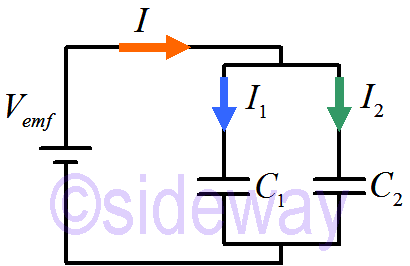When capacitors are in parallel, the effective parallel capacitance is ```𝐼=𝐼1+𝐼2⇒𝑄=𝑄1+𝑄2 ⇒𝐶equivalent𝑉emf=𝐶1𝑉emf+𝐶2𝑉emf ⇒𝐶equivalent=𝐶1+𝐶2``` For capacitor made of the same material and with the same 𝑠, the capacitance follows straight from the definition of capacitance 𝐶=𝜀0𝐴𝑠 ```𝐶equivalent=𝜀0𝐴1𝑠+𝜀0𝐴2𝑠=𝜀0𝑠(𝐴1+𝐴2)=𝜀0𝑠𝐴equivalent ⇒𝐴equivalent=𝐴1+𝐴2 ```

## Source and Reference

ID: 200100502 Last Updated: 1/5/2020 Revision: 0Home 5

Management

HBR 3

Information

Recreation

Culture

Chinese 1097

English 337

Computer

Hardware 154

Software

Application 207

Latex 35

Manim 203

Numeric 19

Programming

Web 285

Unicode 504

HTML 65

CSS 63

SVG 9

ASP.NET 240

OS 422

Python 64

Knowledge

Mathematics

Algebra 84

Geometry 32

Calculus 67

Engineering

Mechanical

Rigid Bodies

Statics 92

Dynamics 37

Control

Natural Sciences

Electric 27